# Analytic mapping

(diff) ← Older revision | Latest revision (diff) | Newer revision → (diff)

analytic morphism

A morphism of analytic spaces considered as ringed spaces (cf. Analytic space; Ringed space). An analytic mapping of a space (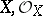) into a space (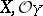) is a pair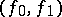, whereis a continuous mapping, while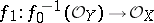is a homomorphism of sheaves of rings on. If the spaces are complex, an analytic mapping is also called a holomorphic mapping.

If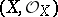and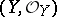are reduced analytic spaces, the homomorphism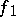is completely determined by the mapping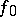and is the inverse mapping of the germs of functions corresponding to. Thus, in this case an analytic mapping is a mappingsuch that for anyand for anyone has.

A fibre of an analytic mapping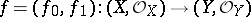at a pointis the analytic subspace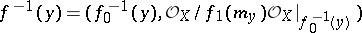of the space, where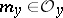is the sheaf of germs of functions that vanish at the point. Putting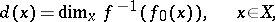one obtains the inequality(*)

Ifand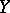are reduced complex spaces, then the set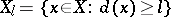is analytic infor any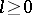.

An analytic mappingis called flat at a pointif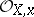is a flat module over the ring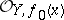. In such a case (*) becomes an equality. An analytic mapping is called flat if it is flat at all points. A flat analytic mapping of complex spaces is open. Conversely, ifis open,is smooth and all fibres are reduced, thenis a flat analytic mapping. The set of points of a complex or a rigid analytic spaceat which an analytic mappingis not flat is analytic in. Ifandare reduced complex spaces, whilehas a countable base, thencontains a dense everywhere-open set over whichis a flat analytic mapping. If an analytic mappingof complex spaces is flat, then the set ofat which the fibre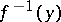is not reduced or normal is analytic in.

Letbe an analytic mapping of reduced complex spaces. If, then there exists a stratificationwhere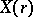are analytic sets andfor large, with the following property: Any pointhas a neighbourhoodinsuch that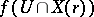is a local analytic set in, all irreducible components of germs of which have dimensionat. Ifis proper, then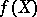is an analytic set in. This is a particular case of the finiteness theorem for analytic mappings.

Let,be complex spaces and letbe compact. Then it is possible to endow the setof all analytic mappingswith the structure of a complex space such that the mapping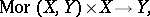which maps the pair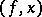into, is analytic. In particular, the group of automorphisms of a compact complex spaceis a complex Lie group, acting analytically on.

How to Cite This Entry:
Analytic mapping. Encyclopedia of Mathematics. URL: http://encyclopediaofmath.org/index.php?title=Analytic_mapping&oldid=12227
This article was adapted from an original article by D.A. Ponomarev (originator), which appeared in Encyclopedia of Mathematics - ISBN 1402006098. See original article